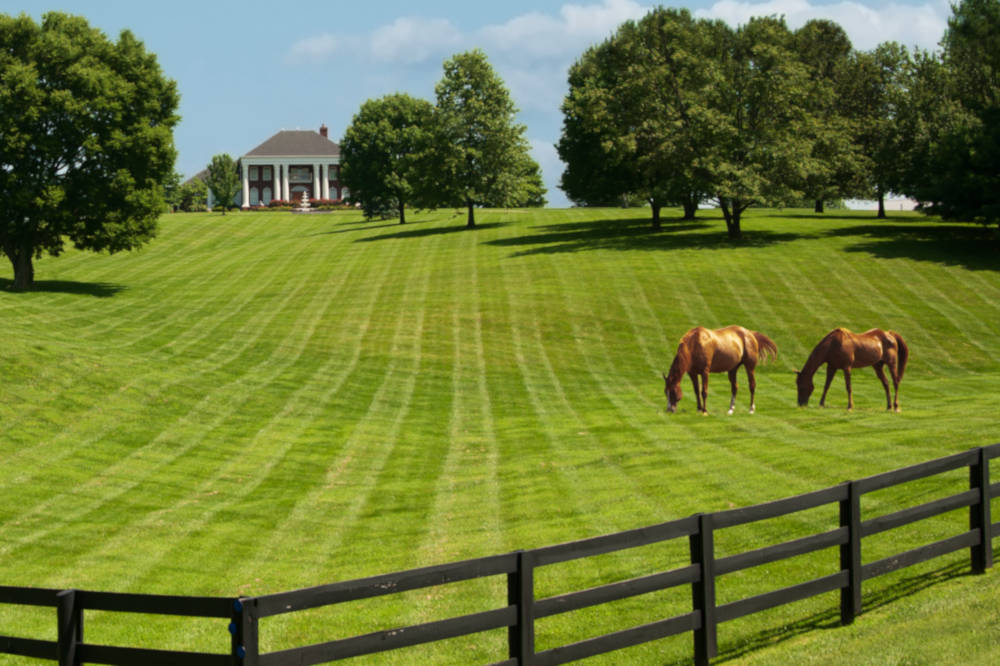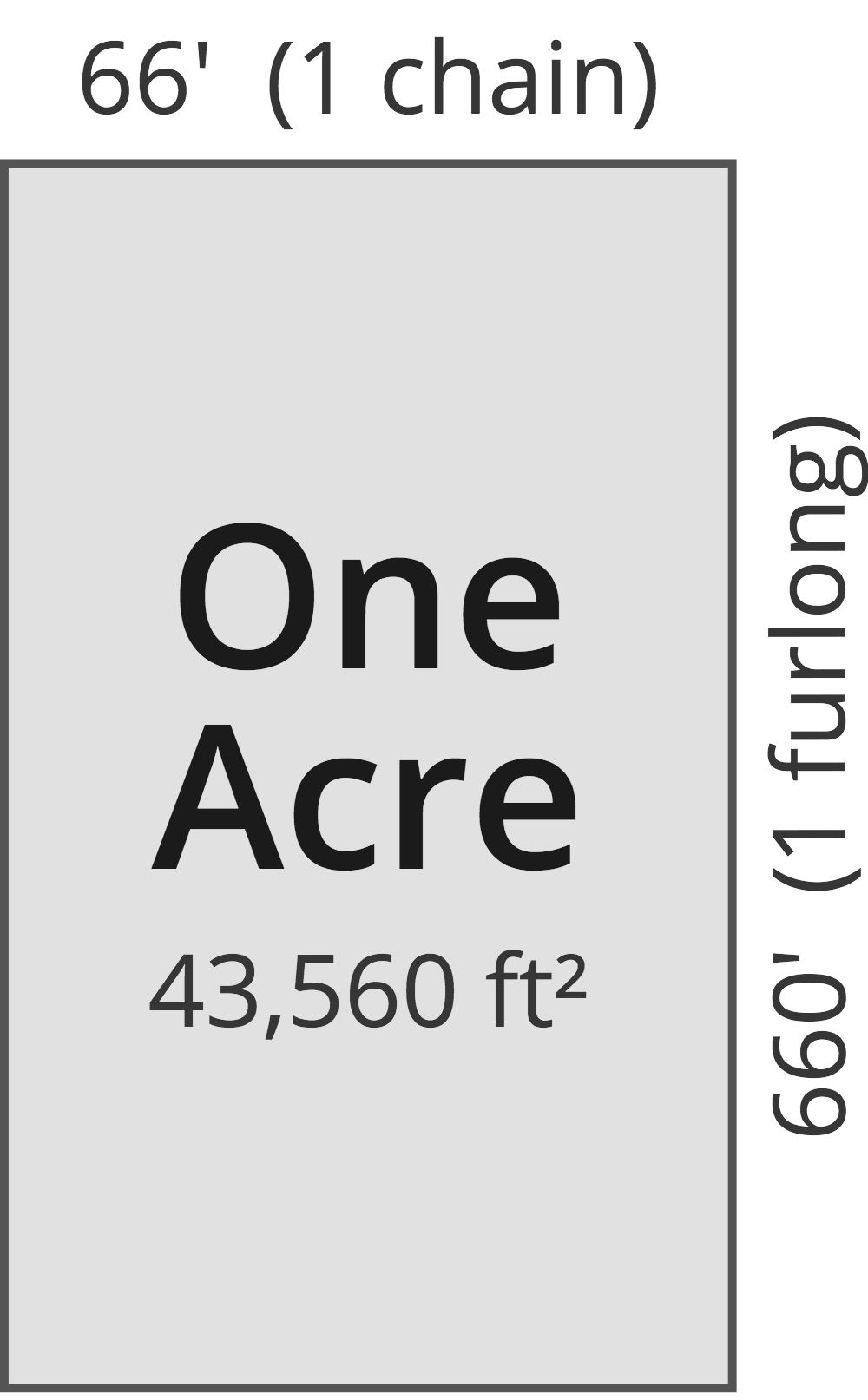# Acreage Calculator – Find Acres Using a Map or Land Dimensions

Calculate an area in acres by entering the length and width. For more complex shapes, use the map to calculate acreage by placing pins on the edges of the land to be measured.

## Total Acreage:

 Acres Hectares Square Feet Square Meters Square Miles Square Kilometers Perimeter in Feet Perimeter in Meters
Learn how we calculated this below

## What is an Acre?

An acre is a measurement of land area used in the Imperial measurement system (U.S. and informally in the U.K. and its former colonies). It was originally the amount of land a yoke of oxen could plow in a day.

Now, it is defined as an area measuring one chain by one furlong — not entirely more useful units either!

We’ll discuss how much an acre is in a more reasonable way below. But, know that an acre is still a common land area measurement used in the U.S., especially in real estate and government land transactions.## How Big is an Acre?

So having a dimension of one chain by one furlong, you might be wondering how big an acre is. One acre is equal to 43,560 square feet, which is just a little bit smaller than a football field and about the same size as a soccer field.

The dimensions of an acre have been historically defined as being equal to an area that is 66 feet (1 chain) by 660 feet (1 furlong).

Defining the size in acreage this way allowed farmers to more easily determine how much land they could plow per day. The furlong was originally the distance an oxen team could plow without rest. So they defined the acre in terms of how many furrows (the length of the furlong) could be plowed in a single day.Outside of land surveys, these units of measure are rarely used. Refer to the table below to see how big an acre is relative to more commonly used measurements.

### Size of One Acre

Here’s a quick reference table showing the size of an acre.

Chart showing the dimensions of one acre in various units of area measurement.
Unit of Measure Dimension Equal to 1 Acre
Hectares 0.4047 hectares
Meters 4,047 m²
Miles 0.0015625 miles² (640 acres = 1 mile²)
Yards 4,840 yds²
Square Feet 43,560 ft²
Square Inches 6,272,640 in²
Perches 160 perches (1 perch = 1 rod)
Rods 160 rod² (1 rod = 16 ½ feet)

You can also use our acreage converter to convert the area in acres to another unit of measure.

## How to Calculate Acres

The easiest way to calculate the number of acres in your land is to use the calculator above. If you know the length and width, simply enter them. The calculator will provide the number of acres, as well as the area in other units.

To calculate acres by hand, multiply your length and width (in feet) to get square feet. Try our length converter if needed. Then divide by 43,560 to determine the size of the land in acres.

Example: Your city house lot is 33′ wide by 102′ long. How many acres do you have?

33′ × 102′ = 3,366 sq ft
3,366 sq ft ÷ 43,560 = 0.077 acres

You can quickly find the square footage of an area using our square footage calculator.

Unfortunately, property is not always divided into perfect squares, and finding the acreage of irregular shapes can be a little more challenging.

The trick to finding the size of these irregular plots in acres is to break down the land into smaller geometric shapes first. Find the size of those smaller spaces separately, and then add them together.

Another great way to find the area of irregular shapes is to use the interactive map above to determine the actual size in acres.

Keep in mind that sometimes property lines can be vague or off by several feet. If you need precise measurements for something like fencing, always measure the land directly and do not go off of reported acreage. While your plot may have been purchased as 1 acre, your actual property lines could make it slightly bigger or smaller depending on how and when it was surveyed.

In addition, when installing things like fences, sometimes trees, large rocks, and other landscaping irregularities will change the path of the fence. Always get precise measurements before purchasing materials.

### Acre Lot Portions

While acreage is the most common method for measuring large plots of land, it’s also sometimes used for home lots as well. The average home is on a ⅓ acre lot, but it’s also common to have a total lot that is ½ or ⅔ acre.

While it’s best to measure the area to get the most accurate measurements, the following chart breaks down partial acres into square footage and the perimeter in linear footage.

Chart showing the dimensions of one acre in various units of area measurement.
Lot Size Area in Square Feet Perimeter in Linear Feet
¼ acre 10,890 sq ft 209 ft
⅓ acre 14,520 sq ft 279 ft
½ acre 21,780 sq ft 418 ft
⅔ acre 29,040 sq ft 558 ft
¾ acre 32,670 sq ft 627 ft

## How to Calculate the Perimeter of an Acre

The calculator above will also give you the perimeter in linear feet, which you’ll need if you plan on fencing in your property, as this is the most common way that fences are sold and installed. The average acre has 835 linear feet around the perimeter for which to fence.

Note that this measurement can vary depending on your exact parcel size. To calculate this by hand, you can measure each of the four sides individually in feet, and add them together.

To calculate the perimeter by hand, you can measure each of the sides individually, then add them together. If you measured in feet, the result will be the perimeter in linear feet.

### Why do we use acres?

Acres have become a common measurement in the US for an area measuring 43,560 sq ft. The unit of measurement came from the number of furrows an oxen could likely plow in one day – about 66, each one measuring 660 feet long.

### How much does 1 acre look like?

An acre is roughly the size of a soccer field or a little smaller than the size of a football field.

### How many houses can fit on 1 acre?

The average home in the US sits on a total of ⅓ of an acre, which would make three homes per acre the average. However, average lot sizes have decreased; the former average size was ½ acre, which would mean two homes on large-sized lots, while homes can sit on smaller lots of ¼ acre comfortably, which would make four homes per acre.

### How many acres make a ranch or farm?

Farm acreage depends on the use and can range from ½ acre for a small farm to 40 acres for a large farm. Ranches may have legal definitions of size by state, with a small range being defined as around 100 acres in most areas.

### How many acres are in a square mile?

There are 640 acres in one square mile.

## References

1. National Institute of Standards and Technology, U.S. Survey Foot: Revised Unit Conversion Factors, https://www.nist.gov/pml/us-surveyfoot/revised-unit-conversion-factors